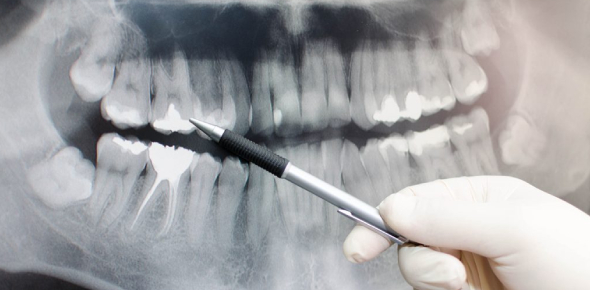# Oral Radiology Examination Practice Test!

14 Questions | Total Attempts: 38589SettingsThis quiz will test the dental student's understanding and knowledge of radiation physics and the fundamentals of radiographic interpretation.

• 1.
An example of particulate radiation is:
• A.

Gamma rays

• B.

X-rays

• C.

Cathode rays

• D.

None of the above

• E.

All of the above

• 2.
• A.

• B.

• C.

• D.

• E.

• 3.
The cathode and anode are parts of an x-ray tube. The anode is negatively charged.
• A.

The first statement is correct. The second statement is wrong

• B.

The first statement is wrong. The second statement is correct

• C.

Both the statements are correct

• D.

Both the statements are wrong

• 4.
One of the following types of radiation has high Linear Energy Transfer (LET) and hence the potential for more radiation related tissue damage:
• A.

Gamma rays

• B.

X-rays

• C.

Alpha rays

• D.

Micro waves

• E.

• 5.
• A.

• B.

• C.

• D.

Mandibular floor of the mouth occlusal

• E.

• 6.
The radiograph shown here is a:
• A.

• B.

• C.

• D.

P-A view of the skull

• E.

A-P view of the skull

• 7.
In the construction of an  x-ray tube, the function of a step- down transformer is to
• A.

Convert the line current of 110 volts to less than 10 milli ampeares

• B.

Convert the line current of 110 volts to less than 10 volts

• C.

Convert the line current of 220 volts to less than 10 volts

• D.

Convert the line current of 110 volts to less than 100 amperes

• 8.
In the radiographic principle, "SLOB rule, "same side movement of the object is ______ and the oppsite side movement of the object is ______. What are the missing words in the same sequence.
• A.

Lateral and buccal

• B.

Lingual and buccal

• C.

Lingual and both sides

• D.

Lateral and both sides

• 9.
70 kilovolts equals
• A.

70,000 volts

• B.

700,000 volts

• C.

70,000,000 volts

• D.

7,000 volts

• E.

700 volts

• 10.
The "rotating anode" design in an x-ray tube limits the amount of
• A.

Lighting at anode

• B.

Heat production at anode

• C.

Electron production at cathode

• D.

Speed of electrons from cathode to anode

• E.

X-rays that are produced at the anode

• 11.
An example of an electro-magnetic radiation is
• A.

Alpha rays

• B.

Beta rays

• C.

Cathode rays

• D.

Gamma rays

• E.

None of the above

• 12.
The sharpness of the image is determined to a large extent by the
• A.

Shape of the object

• B.

The focal spot size

• C.

The make of the x-ray machine

• D.

The distance between the anode and cathode

• E.

None of the above

• 13.
The radiograph shown here is frequently used in Orthodontics for study of the growth and development of the head. Name the radiograph
• A.

PA skull

• B.

AP skull

• C.

Submento vertex view

• D.

Lateral Cephalometric view

• E.

Panoramic view

• 14.
The "p" in kVp stands for
• A.

Percentage

• B.

Parallel

• C.

Punitive

• D.

Peak

• E.

Paired

Related TopicsBack to top